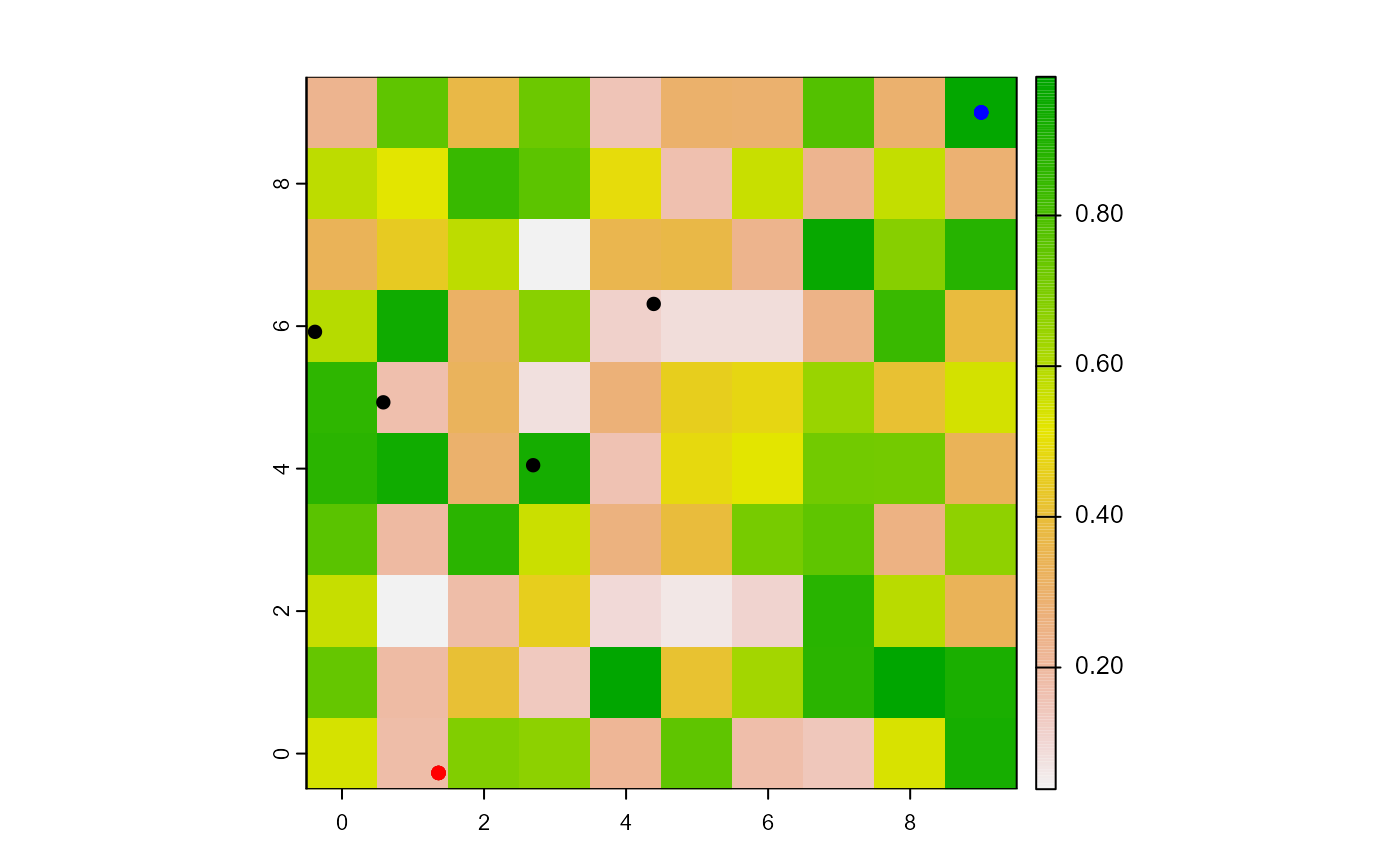Move the turtles to the agents' locations.

moveTo(turtles, agents)

# S4 method for agentMatrix,matrix
moveTo(turtles, agents)

## Arguments

turtles

AgentMatrix object representing the moving agents.

agents

Matrix (ncol = 2) with the first column pxcor and the second column pycor representing the patches coordinates, or

          AgentMatrix object representing the moving agents.

## Value

AgentMatrix representing the turtles with updated coordinates and updated data for their previous coordinates prevX and prevY.

## Details

The number of agents must be equal to 1 or to length turtles.

     The turtle's headings are not affected with this function.

If a turtle is moving to a patch location, it will be located at

the patch center.

Sarah Bauduin

## Examples

w1 <- createWorld(
minPxcor = 0, maxPxcor = 9, minPycor = 0, maxPycor = 9,
data = runif(100)
)
t1 <- createTurtles(n = 5, coords = randomXYcor(w1, n = 5))
plot(w1)
points(t1, col = "black", pch = 16)

t1 <- moveTo(turtles = t1, agents = turtle(t1, who = 0))
points(t1, col = "red", pch = 16)

t1 <- moveTo(turtles = t1, agents = patch(w1, 9, 9))
points(t1, col = "blue", pch = 16)